# Lesson Notes By Weeks and Term - Primary 3

Sequencing and comparing numbers

TERM: 1st Term

WEEK: 3

CLASS: Primary 3

AGE: 8 years

DURATION: 5 periods of 40 minutes each

DATE:

SUBJECT: Mathematics

TOPIC: Sequencing and comparing numbers

SPECIFIC OBJECTIVES: At the end of the lesson, the pupils should be able to

1. Sequence numbers up to 1000
2. Read, represent and compare numbers on a number line

INSTRUCTIONAL TECHNIQUES: Explanation, question and answer, demonstration, practical, assessments

INSTRUCTIONAL MATERIALS: Base 10 kit, 100 number board, scrap paper, videos from source- https://www.youtube.com/watch?v=Uf6rifkw2tE

PERIOD 1: Sequencing and comparing numbers

 PRESENTATION TEACHER’S ACTIVITY PUPIL’S ACTIVITY STEP 1MENTAL MATHS The teacher begins the lesson with some mental calculationsCount in 10s from any multiples of 10 between 300-600 Pupils respond and participate STEP 2CONCEPTDEVELOPMENT The teacher draws a number line on the board missing out some numbers as followsThe pupils are asked to draw the number line in their book and fill out the missing numbers. One of them is asked to solve it on the board The teacher circles the number 601 and asks the pupils to mention numbers smaller than 601(597-600) and bigger than 601(602-607) The teacher then covers the number line and writes numbers 597-607 randomly on the board and asks the pupils to arrange them from the smallest to the greatest and also from the greatest to the smallest The teacher repeats this for 314-323, 267-274, 467-473 The teacher then draws the below number line on the board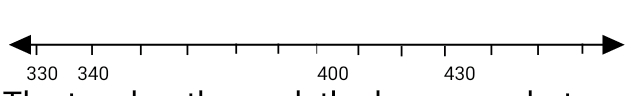The teacher then ask the learners what number will come after 340 which is 350 because the number is going up in tensThe learners are then asked to fill up the remaining missing number The same thing is done for the below number line(which is counting in 5’s)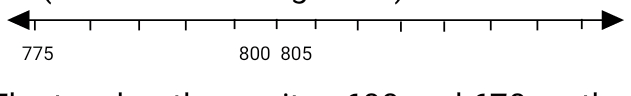The teacher then writes 600 and 670 on the board and asks which is bigger? The learners are then asked to point in the direction the number 600 will go on the number line(which is the left-hand side because 600 is less than 670), she also asks the direction 670 will go on the number line(which is the right hand side because 670 is greater than 600)The teacher repeats this process with other different numbers- 213 and 263, 198 and 214, 345 and 415 Pupils pay attention and participate STEP 3CLASS-WORK 1. Fill in the missing  numbers on the number line2. Can you find these numbers-495, 505, 550,  on the number line? Write them on the number line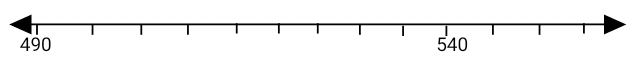3. Which number is the biggest? Show it on the number linePupils attempt their class work STEP 4HOME-WORK 1. Fill in the missing  numbers on the number line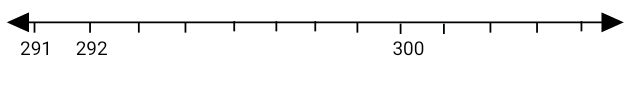2. Can you find these numbers-940, 905, 965, 930, 920, 945, 955, 925, 935, 910, 960 on the number line? Write them on the number line3. Which number is the biggest? Show it on the number linePupils attempt their class work STEP 5SUMMARY The teacher summarizes by ensuring the pupils practice severally on the number line She marks their class works, makes corrections where necessary and commends them positively

PERIOD 2: Comparing, ordering and rounding off numbers

 PRESENTATION TEACHER’S ACTIVITY PUPIL’S ACTIVITY STEP 1MENTAL MATHS The teacher begins the lesson with some mental calculationsWhat is 100 more than1. 1512. 3433. 7774. 4635. 148 What is 100 less than6. 8377. 4718. 5409. 22310. 654 Pupils respond and participate STEP 2CONCEPTDEVELOPMENT The teacher writes two numbers on the board: 429 and 392 and ask the learners to use pictorials to represent both numbers on the board(squares as hundreds, lines as tens and dots as ones)429- 4 squares 2 lines and 9 dots392- 3 squares 9 lines and 2 dotsThe teacher then explains that 429 is bigger than 392 because  392 has 3 hundreds while 429 has 4 hundreds and 4 hundreds is bigger than 3 hundreds The teacher also asks the pupils to use the base ten kit to represent both numbers(to help visualize the difference in size between the two number)The teacher then explains that we can show that 429 is bigger than 392 by putting in a greater than sign: 429 > 392 The same processes is repeated for 327 and 372(where it is shown that even though both numbers have 3 hundreds, the tens digit in 327 is 2 while the tens digit in 372 is 7 which means 327 is smaller than 372). We an show that 327 is smaller than 372 by putting in a less than sign: 327 <  372 CLASS ACTIVITYThe teacher then writes 289 and 302 on the board and ask the pupils to use the method she just explained to compare both numbers. The same is applied to 309 and 352 The teacher then draws the below number line on the board and asks the pupils to circle 114She then asks the pupils which number is closer to114, 110 or 120. She explains that looking at the number line, It is obvious that 114 is closer to 110.With this, she explains that rounding off means to find the multiple of 10 which is closer to the given numbers For 112, it is closer to 110 so we round it off to 110For 117, it is closer to 120 so we round it off to 120As a rule, 115 is round off to 120 Pupils pay attention and participate STEP 3CLASS-WORK 1. Fill in > or or

PERIOD 3: More numbers up to 1000

 PRESENTATION TEACHER’S ACTIVITY PUPIL’S ACTIVITY STEP 1MENTAL MATHS The teacher begins the lesson with some mental calculationsCount forwards and backwards in 10s from any multiples of 10 between 700 and 1000 Pupils respond and participate STEP 2CONCEPTDEVELOPMENT The teacher draws 342 on the board in simplified pictorials(3 squares, 4 lines and 2 dots) and ask the learners to state how many hundreds, tens and ones are there(3 hundreds, 4 tens and 2 ones) The teacher asks the pupils to put these numbers- 356, 712, 834 in simplified pictorial forms in their note books. She also asks them to write the names of each numbers by the side The teacher then ask the pupils how many tens are in 270(27 tens)She further asks how else the number 270 could be expressed(which is 2 hundreds, 7 tens and 0 ones)The process is repeated for the numbers 520, 140, 330 CLASS ACTIVITYThe teacher draws the following number line on the board and ask the pupils to fill in the missing numbersPupils pay attention and participate STEP 3CLASS-WORK 1 Write the number on the line.a 5 hundreds, 3 tens and 2 ones _______________b 7 hundreds, 5 tens and 6 ones _______________c 1 hundred, 0 tens and 4 ones _______________d 4 hundreds, 9 tens and 5 ones _______________e 6 hundreds, 7 tens and 0 ones _______________ 2 Complete the following:There are _____ tens in 520There are _____ tens in 470There are _____tens in 610There are 84 tens in _____There are 39 tens in _____There are 75 tens in _____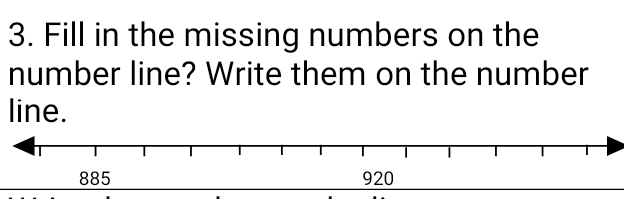Pupils attempt their class work STEP 4HOME-WORK Write the number on the line.a 3 hundreds, 0 tens and 2 ones _______________b 9 hundreds, 6 tens and 0 ones _______________c 5 hundreds, 5 tens and 5 ones _______________d 7 hundreds, 2 ten and 0 ones _______________ The pupils writes it in their homework book STEP 5SUMMARY The teacher summarizes allowing the pupils more opportunities to practice the concepts discussed She marks the class work, make corrections where necessary and commends the pupils positively

PERIOD 4: Numbers up to 1000

 PRESENTATION TEACHER’S ACTIVITY PUPIL’S ACTIVITY STEP 1ORALASSESSMENTS The teacher asks questions on numbers up to 1000 treated so far Pupils respond and participate STEP 2DISCUSSION The teacher discusses all the methods used by some learners in the oral assessments(some of the questions are solved on the board by the learners) and addresses any misconceptions that may have risen Pupils pay attention and participate STEP 3WRITTEN ASSESSMENTS 1 Draw number lines to help you round off the following numbers to the nearestten:a 761 ____          b 927 ____c 484 ____          d 689 ____ 2 Write these numbers from the smallest to the biggest: 145, 457, 45 3 Write the following numbers from biggest to smallest:130, 310, 301 4 Fill in the missing numbers on the number line:5 Fill in > or <:a 751 (  ) 571           b 498 (  ) 488c 561 (  ) 651           d 204 (  ) 240 Pupils attempt their class work STEP 4SUMMARY The teacher marks the written assessments, corrects were necessary and commends the pupils

PERIOD 5: Weekly Test/consolidations

TEACHER’S ACTIVITY: The teacher revises all the concepts treated from period 1-4 and gives the pupils follow through exercises, quiz and tests . She marks the exercises, makes corrections and commends the pupils positively.

PUPIL’S ACTIVITY: The pupils work on the worksheets and exercises given by the teacher individually

ASSESSMENT

1 Write the number:

a 9 hundreds, 1 ten and 7 ones _______________

b 6 hundreds, 8 tens and 2 ones _______________

c 3 hundreds, 3 tens and 0 ones _______________

d 2 hundreds, 0 tens and 3 ones _______________

e 7 hundreds, 2 tens and 9 ones _______________

2 Fill in the missing numbers on the number line: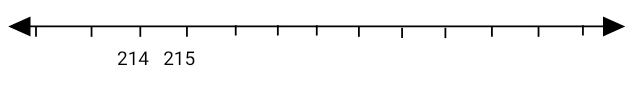3. Can you find these numbers on the number line? Write them on the number line(430, 340, 410, 390, 440, 320, 400, 420, 350, 380, 3304 Fill in > or <:

a 489 (   ) 849

b 325 (   ) 352

c 674 (   ) 664

d 299 (   ) 289

e 851 (   ) 951

5 Draw number lines to help you round off the following numbers to the nearest ten:

a 994 ____

b 677 ____

c 258 ____

d 422 ____

e 571 ____

f 125____